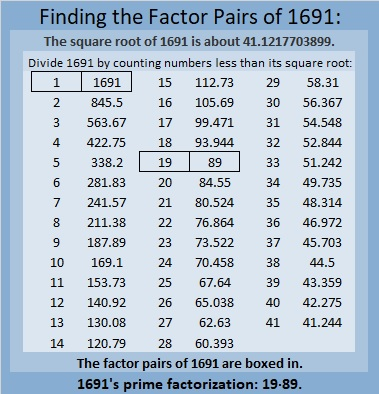1691 Jack 0’Lantern Time

Contents

Today’s Puzzle:

This smiling Jack O’Lantern actually has a few tricks up his sleeve. Don’t let yourself get fooled by any of them. Using logic, write the numbers from 1 to 12 in the first column as well as in the top row so that those numbers and the given clues can be a multiplication table.

Have a safe and happy Halloween!Here’s the same puzzle with just the clues:Factors of 1691:

• 1691 is a composite number.
• Prime factorization: 1691 = 19 × 89.
• 1691 has no exponents greater than 1 in its prime factorization, so √1691 cannot be simplified.
• The exponents in the prime factorization are 1 and 1. Adding one to each exponent and multiplying we get (1 + 1)(1 + 1) = 2 × 2 = 4. Therefore 1691 has exactly 4 factors.
• The factors of 1691 are outlined with their factor pair partners in the graphic below.